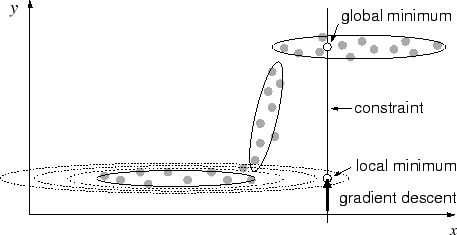Next: 4.2 Recall algorithm Up: 4.1 Motivation Previous: 4.1.1 Why abstract recurrent

## 4.1.2 Potential fields and local minima

For the mixture model, to obtain a potential field, the following two possibilities exist (they are not exclusive): use the negative of the probability density function (the sum of Gaussian functions), or use the sum of inverse Mahalanobis distances over all units (equivalent to a sum over Coulomb potentials (Bachmann et al., 1987)).

Given a potential field, a functional mapping from an input to an output can be achieved by defining a constrained subspace whose offset from zero is given by the input. Then, the completion of the input, which yields the output in its components, can be gained by finding a point on the constraint that has a minimal potential value. A gradient descent along the constraint may be used to find this minimum.

However, this gradient descent may end in a local minimum (figure 4.1). For both the negative density and the inverse-Mahalanobis potential, a potential valley corresponding to an ellipsoid may extend along the tips of the ellipsoid toward the constraint. At the intersection, this valley results in a local minimum within the constrained space. Such a local minimum may be far away from the distribution of training patterns and must therefore be avoided. Thus, instead of computing a gradient descent in a potential field, we directly compute the point on the constraint that is closest to the closest ellipsoid.Next: 4.2 Recall algorithm Up: 4.1 Motivation Previous: 4.1.1 Why abstract recurrent
Heiko Hoffmann
2005-03-22